Try NerdPal! Our new app on iOS and Android

# Expand the logarithmic expression $\ln\left(2\cdot e\right)$

## Related Videos

Go!
Go!
1
2
3
4
5
6
7
8
9
0
a
b
c
d
f
g
m
n
u
v
w
x
y
z
.
(◻)
+
-
×
◻/◻
/
÷
2

e
π
ln
log
log
lim
d/dx
Dx
|◻|
θ
=
>
<
>=
<=
sin
cos
tan
cot
sec
csc

asin
acos
atan
acot
asec
acsc

sinh
cosh
tanh
coth
sech
csch

asinh
acosh
atanh
acoth
asech
acsch

### Videos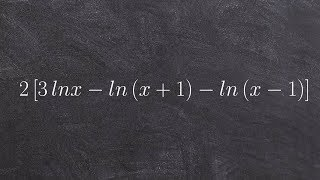### Pre-Calculus - Condensing a logarithmic expression to one logarithm 2[3lnx - ln(x+1)-ln(x-1)]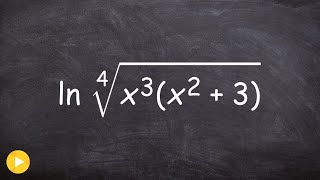### Expanding a natural logarithmic expression### Master Expanding Logarithmic Expressions using the rules of logarithms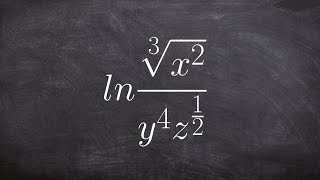### Tutorial - Expanding logarithmic expressions ex 15, ln (cuberoot(x^(2/3)) / (y^4 z^1/2))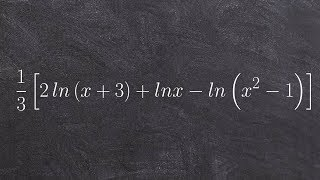### Tutorial - Condensing logarithmic expressions ex 12, 1/3(2ln(x+3)+lnx-ln(x^2-1))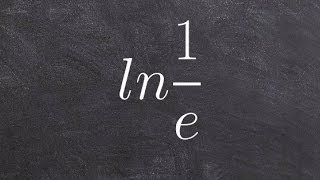SnapXam A2

### beta Got another answer? Verify it!

Go!
1
2
3
4
5
6
7
8
9
0
a
b
c
d
f
g
m
n
u
v
w
x
y
z
.
(◻)
+
-
×
◻/◻
/
÷
2

e
π
ln
log
log
lim
d/dx
Dx
|◻|
θ
=
>
<
>=
<=
sin
cos
tan
cot
sec
csc

asin
acos
atan
acot
asec
acsc

sinh
cosh
tanh
coth
sech
csch

asinh
acosh
atanh
acoth
asech
acsch

$\ln\left(2\cdote\right)$